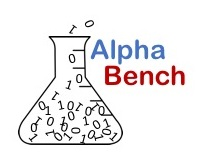# Simple Regression Techniques with Python and NumPy

Complete overview of simple regression techniques using Python and NumPy. Learn how to calculate and graph best fit lines with NumPy, matplotlib and seaborn.

Linear regression is one of the most used modeling techniques across multiple domains. This tutorial covers regression techniques on stock prices, but are easily applied in many settings.